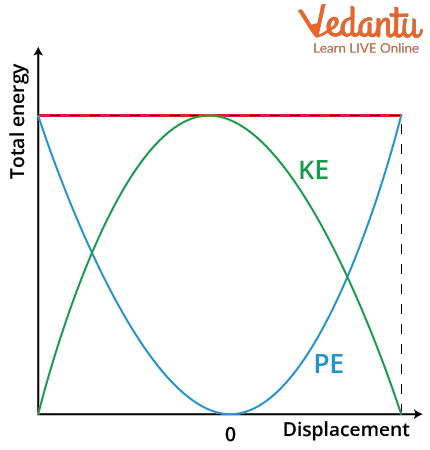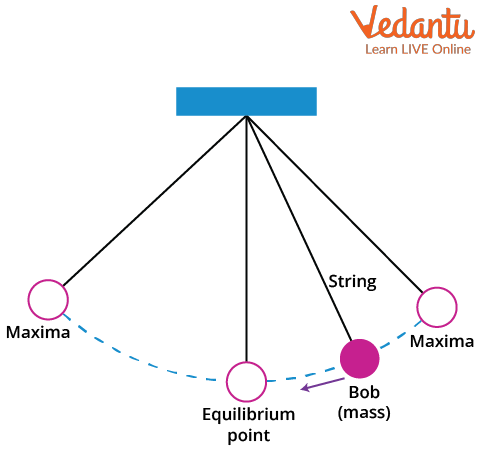Courses
Courses for Kids
Free study material
Offline Centres
More

# Energy in SHM and Its Frequency of Variation for JEELast updated date: 02nd Dec 2023
Total views: 21.3k
Views today: 0.21k## An Introduction to Energy in SHM

Simple harmonic motion is nothing but a to and fro motion of an object. Take for an example the pendulum of a clock that moves between two extreme points from its mean point. Thus, we can say that simple harmonic motions are oscillatory and periodic as they repeat the motion after a certain period of time. However, all oscillatory movements cannot be simple harmonic motions. A simple harmonic motion has two energies: potential energy and kinetic energy. Take the earlier example of a pendulum of a clock swinging, whose velocity is maximum at the equilibrium or mean position, thus the kinetic energy at the point is also maximum.

## Examples of Simple Harmonic Motion or SHM

The oscillatory and periodic motion of an object is called simple harmonic motion. There are several examples of a simple harmonic motion that we encounter in our day-to-day life. Some of these include the following:

1. Bungee Jumping

The bungee cord, when released, allows the person to move to and fro or up and down which is a good example of SHM.

Even with a single push, a cradle can move to and fro for hours which entails SHM.

1. A Simple Pendulum

The to and fro motion of the pendulum of a clock is a perfect example of a simple harmonic motion.

1. A Swing

The motion is pretty understandable. While pushing the swing, we witness the motion to and fro motion, which is another example of SHM.

## Kinetic Energy of SHM

As a simple harmonic motion consists of two energies, i.e., potential and kinetic energy, let us derive an expression for the kinetic energy of SHM. The motion of a pendulum or a mass on a spring both can be realised as simple harmonic motion. The motion of the example listed above is somewhat sinusoidal. Thus, if we have an object of mass m, with a velocity v, we know the kinetic energy or KE for such an object can be given as:

$K . E=\dfrac{1}{2} m v^{2}$

Also, the time period for SHM remains constant. Thus, we assume it to be T. A is the amplitude or the distance of the object from the mean to the extreme position. The position of this object at an instant of time is denoted as $x(t)=A \cos (\omega t+\theta)$ where $\theta$ is the phase shift and $\omega=\dfrac{2 \pi}{T}$ is the angular frequency.

We can calculate the velocity of this object by differentiating the equation above where we get

$v=-A \omega \sin (\omega t+\theta)$

Substituting in $\dfrac{1}{2} m v^{2}$, we get,

\begin{align} &K . E=\dfrac{1}{2} m(-A \omega \sin (\omega t+\theta))^{2} \\ &K \cdot E=\dfrac{1}{2} m \omega^{2} A^{2} \sin ^{2}(\omega t+\theta) \\ &K . E=\dfrac{1}{2} k A^{2} \sin ^{2}(\omega t+\theta)\left(\because k=m \omega^{2}\right) \end{align}

For simplicity, let us assume $\theta=0$, $v=-A \omega \sin (\omega t)$

Substituting the value of $v$ in the above equation, we get,

\begin{align} &K . E=\dfrac{1}{2} m(-A w \sin (w t))^{2} \\ &K . E=\dfrac{1}{2} m A \omega^{2} \sin ^{2}(\omega t) \end{align}

## Potential Energy in SHM

We know that the objects that have conservative forces are the ones that have a potential energy. Conservative forces are the forces that are dependent on the initial and final position of an object. It is independent of the path taken by the object. Additionally, in a closed path, work done by a conservative force is zero.

F=-kx is a spring force that is also a conservative force. The potential energy associated with it is  $P \cdot E=\dfrac{1}{2} k x^{2}$

Now, when the object is displaced from its equilibrium position to their mean position, the work done by their restoring force equals:

\begin{align} &d w=-F \cdot d x \\ &d w=-(-k x) d x \\ &w=\int_{0}^{x} k x \cdot d x \\ &w=k \int_{0}^{x} x d x \\ &w=\dfrac{k x^{2}}{2} \\ &w=\dfrac{1}{2} m \omega^{2} x^{2} \end{align}

Thus, the work done by the restoring force equals the potential energy.

$P . E=\dfrac{1}{2} m \omega^{2} x^{2}$

The Potential Energy in the SHM formula can thus be given as $P . E=\dfrac{1}{2} m \omega^{2} x^{2}$ or

\begin{align} &P \cdot E=\dfrac{1}{2} m \omega^{2}(A \cos (w t+\theta))^{2} \\ &P \cdot E=\dfrac{1}{2} k A^{2} \cos ^{2}(w t+\theta) \end{align}

## Total Energy in SHM

The sum total of energy in SHM or single harmonic motion is given as the sum total of potential energy and kinetic energy.

Therefore, T.E=KE+P.E

Substituting the K.E and P.E values derived above, we get,

\begin{align} &P . E=\dfrac{1}{2} k A^{2} \cos ^{2}(w t+\theta) \\ &K . E=\dfrac{1}{2} k A^{2} \sin ^{2}(\omega t+\theta) \\ &T . E=\dfrac{1}{2} k A^{2} \cos ^{2}(w t+0)+\dfrac{1}{2} k A^{2} \sin ^{2}(w t+0) \\ &T . E=\dfrac{1}{2} k A^{2}\left(\cos ^{2}(w t+\theta)+\sin ^{2}(w t+\theta)\right) \\ &\because \text {using trignometric identity } \cos ^{2} \theta+\sin ^{2} \theta=1 \\ &\therefore \text { T.E }=\dfrac{1}{2} k A^{2} \text { or T.E }=\dfrac{1}{2} m \omega^{2} A^{2} \end{align}

Both kinetic and potential energy are always positive.Graph of Total Energy for SHM

## Frequency of Variation of Kinetic Energy

We derived the kinetic energy of a single harmonic motion to be

$K . E=\dfrac{1}{2} m A \omega^{2} \sin ^{2}(\omega t)$

Expanding this equation further we get,

\begin{align} &\Rightarrow \dfrac{1}{2} m A \omega^{2}\left(\dfrac{1-\cos (2 \omega t)}{2}\right) \\ &\because \cos (2 w t)=1-2 \sin ^{2}(w t) \\ &\Rightarrow \dfrac{1}{4} m A w^{2}-\dfrac{1}{4} m A w^{2} \cos (2 \omega t)\end{align}

Thus, the frequency of variation of kinetic energy is $2\omega$ or 2f. Pictorially, this can be represented as shown below. It can be seen from the figure below that at one time the pendulum has a kinetic energy ranging between zero and maximum twice. Thus, the frequency of variation of kinetic energy is twice as that of simple harmonic motion or SHM.Pendulum of a Clock

## Conclusion

A simple harmonic motion is defined as the linear displacement of an object between two extreme points. If the displacement of this object varies as $A~\cos \left(\omega~t+\theta\right)$ from the origin, the motion can be called a simple harmonic motion. The above equation clearly indicates that when the displacement of the object is sinusoidal, the motion is a simple harmonic motion.

A uniform circular motion can also be called a simple harmonic motion. Tie a ball at the end of the string and try to make it move in a horizontal plane with a fixed point. If we view this motion of the ball from the front or sideways, it looks like a to and from motion. As it has motion, it will also have energies associated with it. The energy of a simple harmonic motion is the sum total of potential and kinetic energy. The total energy is the sum total of the two energies which has been derived above.

## FAQs on Energy in SHM and Its Frequency of Variation for JEE

1. Can a motion be oscillatory but not simple harmonic? Explain using an example.

Yes, the motion of any object can be oscillatory but not necessarily simple harmonic. Consider a ball falling from the top of a tower to a perfectly elastic surface. The motion of the ball in this case will be oscillatory and the value of the restoring force will be equal to F = mg unlike F being proportional to -y. Thus, we can conclude that the motion of an object falling from a height on a perfectly elastic surface will be oscillatory but not a harmonic motion.

2. What is the zero point energy of the simple harmonic oscillator?

A harmonic oscillator is a system that has a restoring force equal to the displacement when the system displaces from the equilibrium position. This restoring force can be denoted as F=-kx, where k is a constant that is always positive.

Now, if the force acting on a system is only a single force as this, we call the system a simple harmonic oscillator, whereas when the frictional force is also present, the system is called a damped harmonic oscillator. The zero point energy of a simple harmonic oscillator or SHM is the lowest level of energy that an oscillator has. It can also be seen as an accommodation of minimising the potential and kinetic energy at the same time. However, it cannot be done simultaneously.